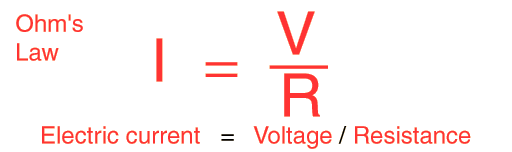# Ohm's Law

For many conductors of electricity, the electric current which will flow through them is directly proportional to the voltage applied to them. When a microscopic view of Ohm's law is taken, it is found to depend upon the fact that the drift velocity of charges through the material is proportional to the electric field in the conductor. The ratio of voltage to current is called the resistance, and if the ratio is constant over a wide range of voltages, the material is said to be an "ohmic" material. If the material can be characterized by such a resistance, then the current can be predicted from the relationship:Data can be entered into any of the boxes below. Specifying any two of the quantities determines the third. After you have entered values for two, click on the text representing the third in the active illustration above to calculate its value.

Amperes = volts / ohms
 AC version of Ohm's law
Index

DC Circuits

 HyperPhysics***** Electricity and Magnetism R Nave
Go Back

# Voltage Law

The voltage changes around any closed loop must sum to zero. No matter what path you take through an electric circuit, if you return to your starting point you must measure the same voltage, constraining the net change around the loop to be zero. Since voltage is electric potential energy per unit charge, the voltage law can be seen to be a consequence of conservation of energy.

The voltage law has great practical utility in the analysis of electric circuits. It is used in conjunction with the current law in many circuit analysis tasks.The voltage law is one of the main tools for the analysis of electric circuits, along with Ohm's Law, the current law and the power relationship. Applying the voltage law to the above circuits along with Ohm's law and the rules for combining resistors gives the numbers shown below. The determining of the voltages and currents associated with a particular circuit along with the power allows you to completely describe the electrical state of a direct current circuit.Current law Resistor combinations
 Analogy in water circuit
Index

DC Circuits

 HyperPhysics***** Electricity and Magnetism R Nave
Go Back

# Current Law

The electric current in amperes that flows into any junction in an electric circuit is equal to the current which flows out. This can be seen to be just a statement of conservation of charge. Since you do not lose any charge during the flow process around the circuit, the total current in any cross-section of the circuit is the same. Along with the voltage law, this law is a powerful tool for the analysis of electric circuits.The current law is one of the main tools for the analysis of electric circuits, along with Ohm's Law, the voltage law and the power relationship. Applying the current law to the above circuits along with Ohm's law and the rules for combining resistors gives the numbers shown below. The determining of the voltages and currents associated with a particular circuit along with the power allows you to completely describe the electrical state of a direct current circuit.Voltage law Resistor combinations
 Analogy in water circuit
Index

DC Circuits

 HyperPhysics***** Electricity and Magnetism R Nave
Go Back